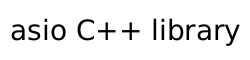### require_concept

A customisation point that applies a concept-enforcing property to an object.

```constexpr unspecified require_concept = unspecified;
```

The name `require_concept` denotes a customization point object. The expression ```asio::require_concept(E, P)``` for some subexpressions `E` and `P` (with types `T = decay_t<decltype(E)>` and ```Prop = decay_t<decltype(P)>```) is expression-equivalent to:

• If ```is_applicable_property_v<T, Prop> && Prop::is_requirable_concept``` is not a well-formed constant expression with value `true`, `asio::require_concept(E, P)` is ill-formed.
• Otherwise, `E` if the expression `Prop::template static_query_v<T> == Prop::value()` is a well-formed constant expression with value `true`.
• Otherwise, `(E).require_concept(P)` if the expression `(E).require_concept(P)` is well-formed.
• Otherwise, `require_concept(E, P)` if the expression `require_concept(E, P)` is a valid expression with overload resolution performed in a context that does not include the declaration of the `require_concept` customization point object.
• Otherwise, `asio::require_concept(E, P)` is ill-formed.
##### Requirements

Header: `asio/require_concept.hpp`

Convenience header: `asio.hpp`